Скачать презентацию Turbulence Energetics in Stably Stratified Atmospheric Flows Sergej

• Количество слайдов: 25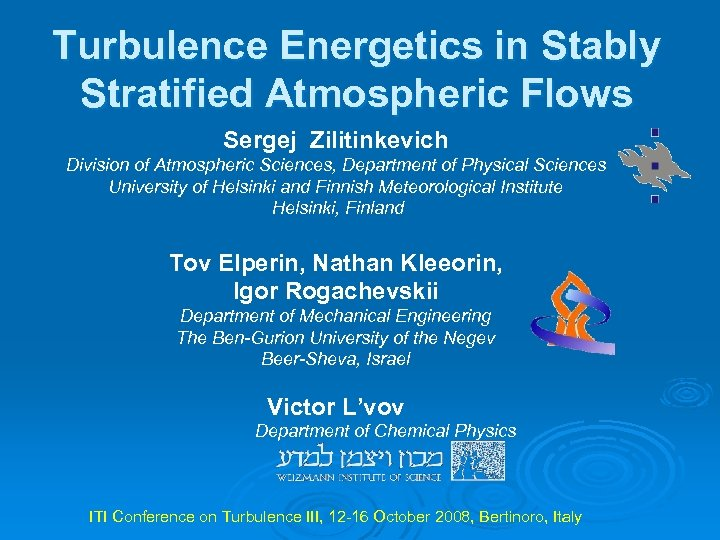Turbulence Energetics in Stably Stratified Atmospheric Flows Sergej Zilitinkevich Division of Atmospheric Sciences, Department of Physical Sciences University of Helsinki and Finnish Meteorological Institute Helsinki, Finland Tov Elperin, Nathan Kleeorin, Igor Rogachevskii Department of Mechanical Engineering The Ben-Gurion University of the Negev Beer-Sheva, Israel Victor L’vov Department of Chemical Physics ITI Conference on Turbulence III, 12 -16 October 2008, Bertinoro, Italy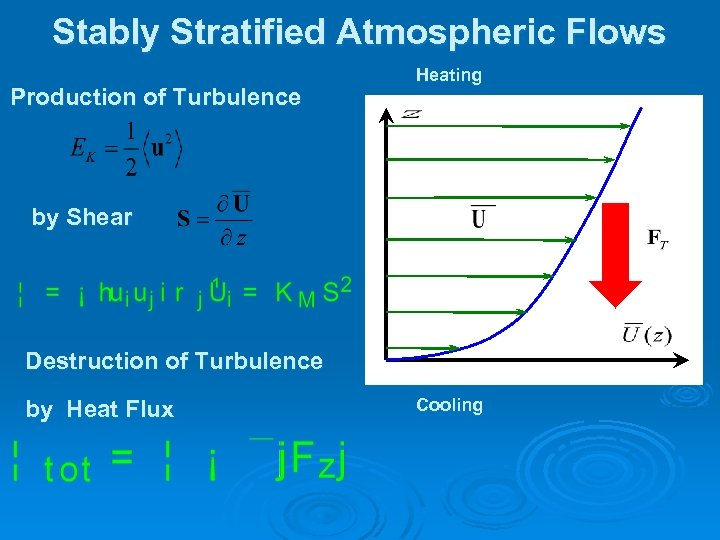Stably Stratified Atmospheric Flows Production of Turbulence Heating by Shear Destruction of Turbulence by Heat Flux Cooling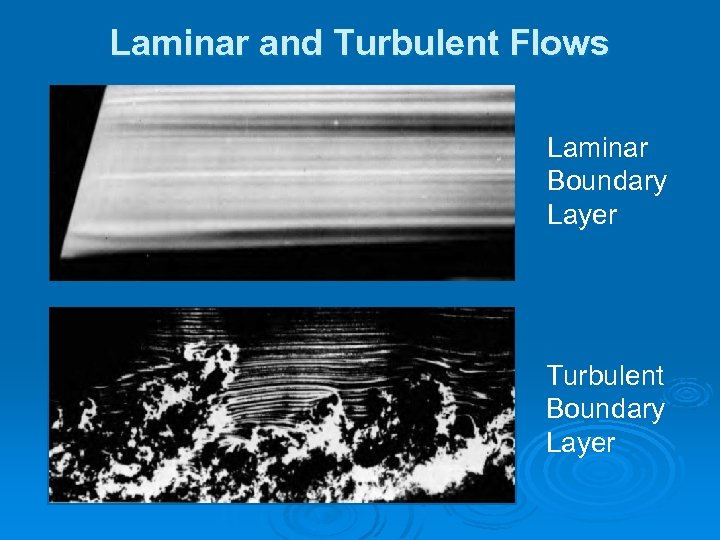Laminar and Turbulent Flows Laminar Boundary Layer Turbulent Boundary Layer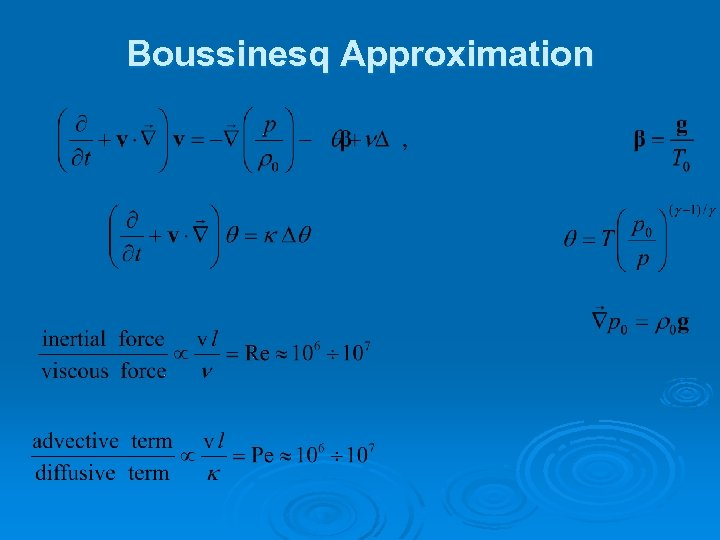Boussinesq Approximation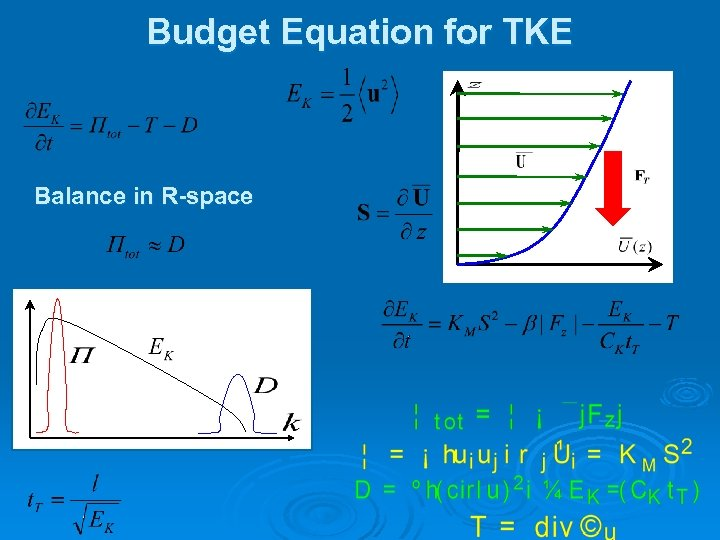Budget Equation for TKE Balance in R-space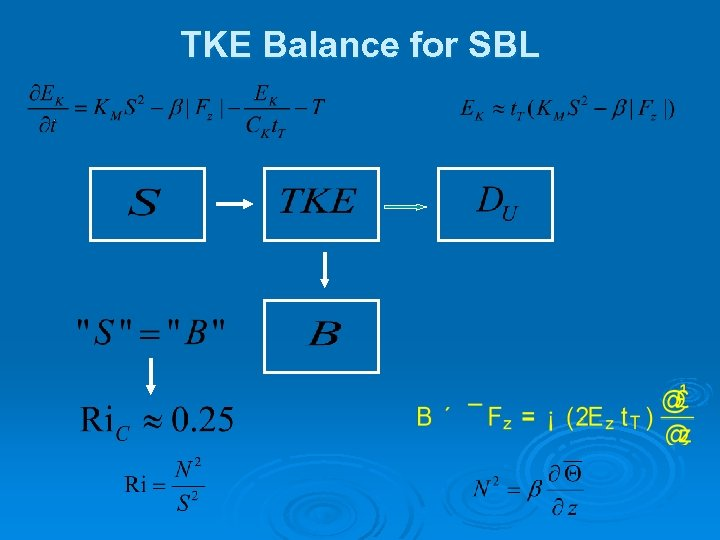TKE Balance for SBL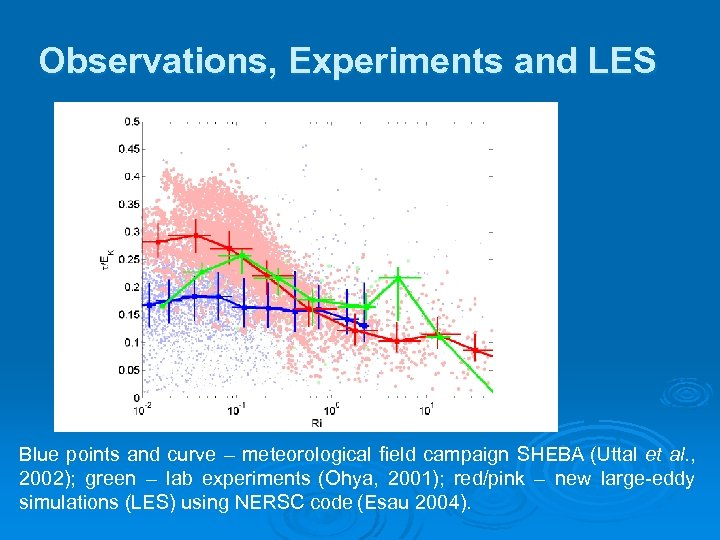Observations, Experiments and LES Blue points and curve – meteorological field campaign SHEBA (Uttal et al. , 2002); green – lab experiments (Ohya, 2001); red/pink – new large-eddy simulations (LES) using NERSC code (Esau 2004).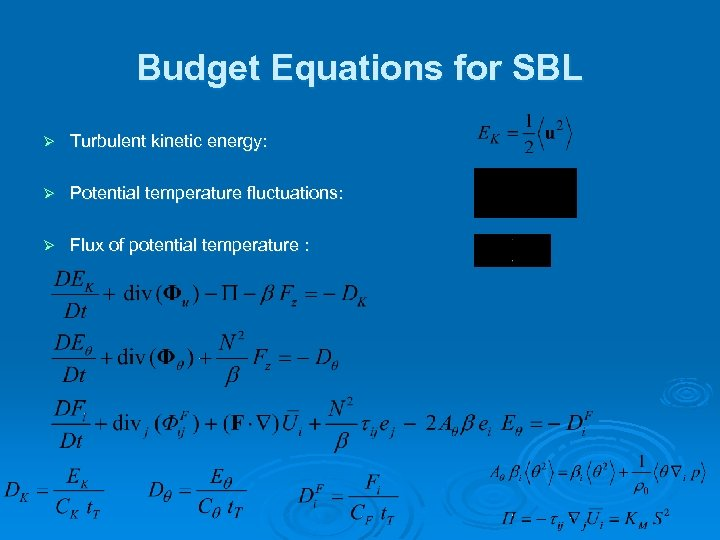Budget Equations for SBL Ø Turbulent kinetic energy: Ø Potential temperature fluctuations: Ø Flux of potential temperature :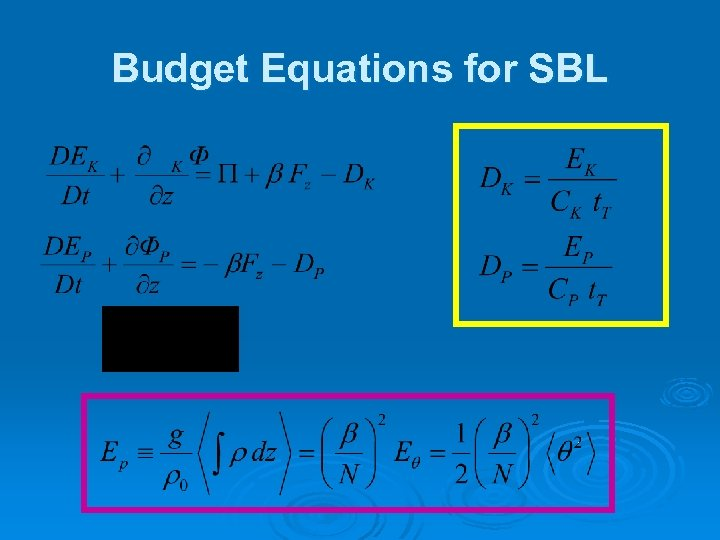Budget Equations for SBL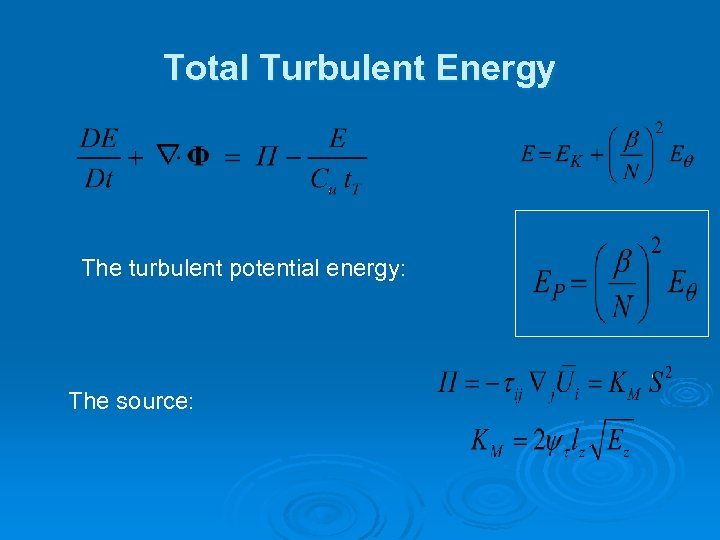Total Turbulent Energy The turbulent potential energy: The source: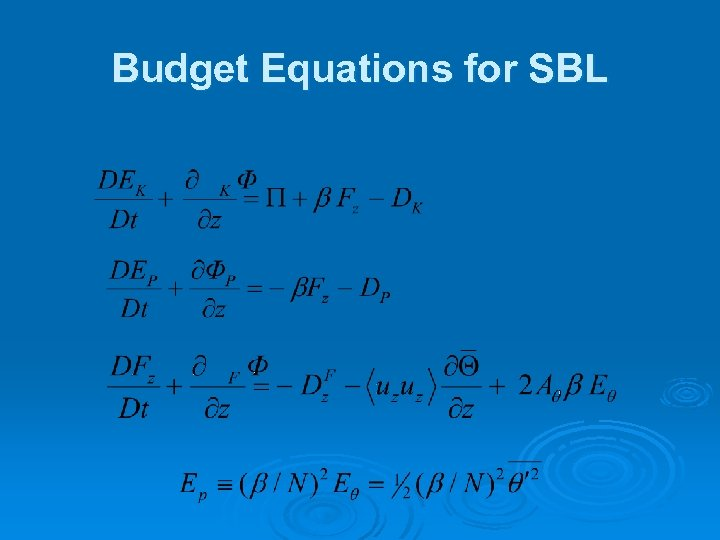Budget Equations for SBL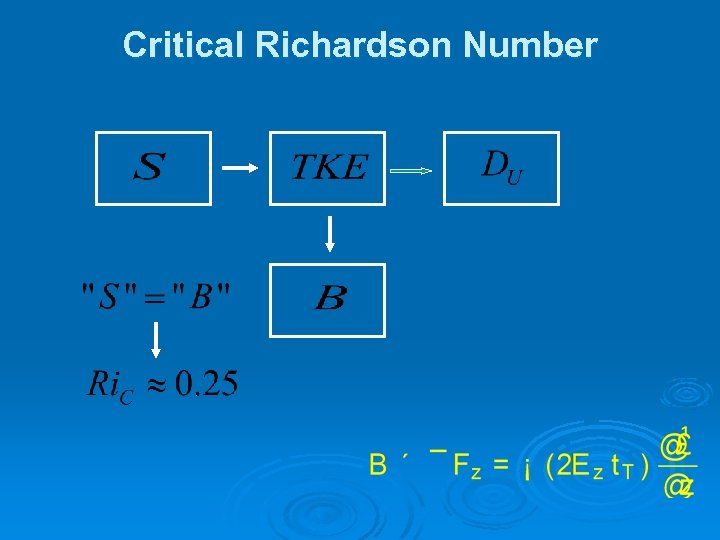Critical Richardson Number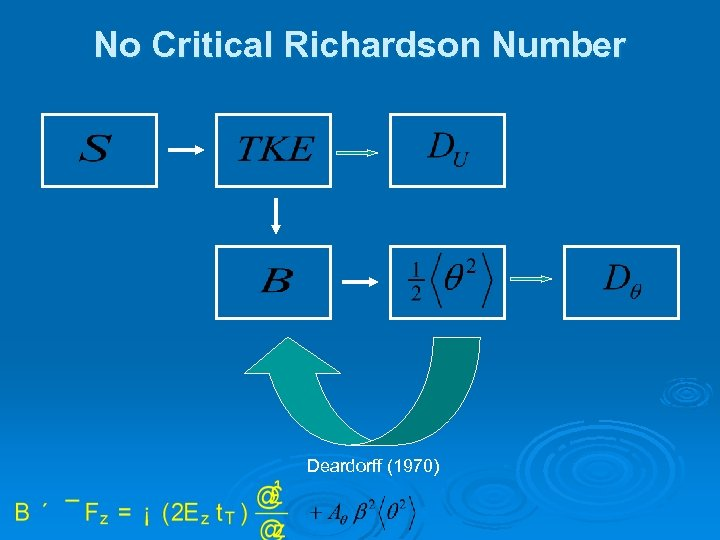No Critical Richardson Number Deardorff (1970)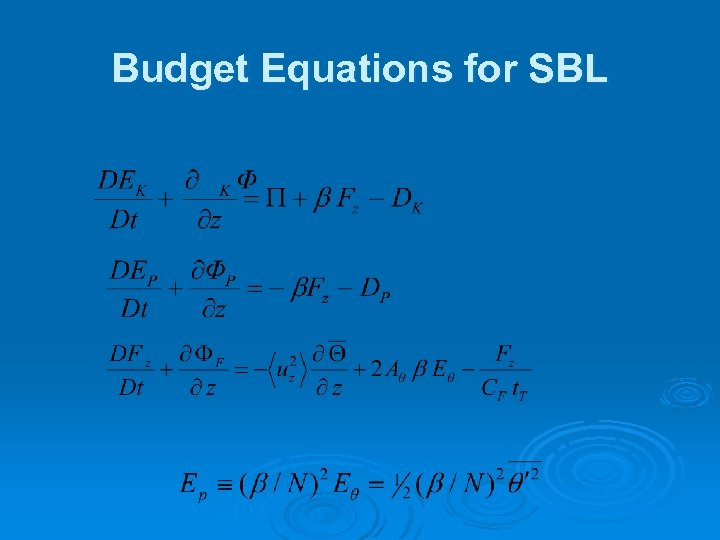Budget Equations for SBL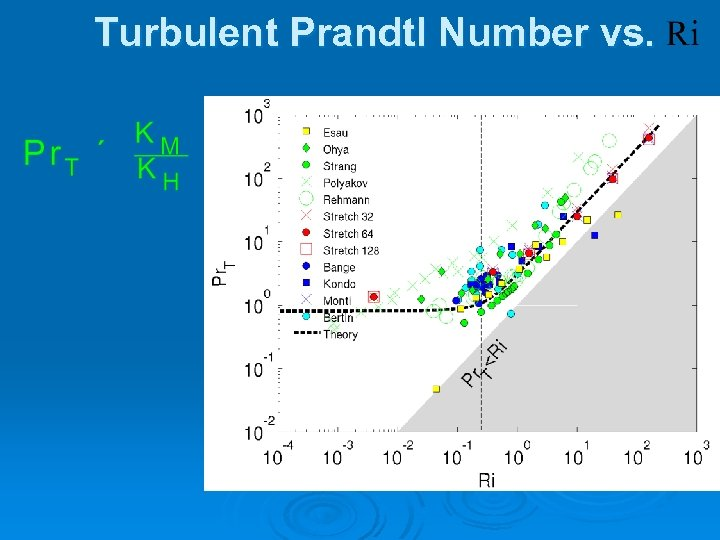Turbulent Prandtl Number vs.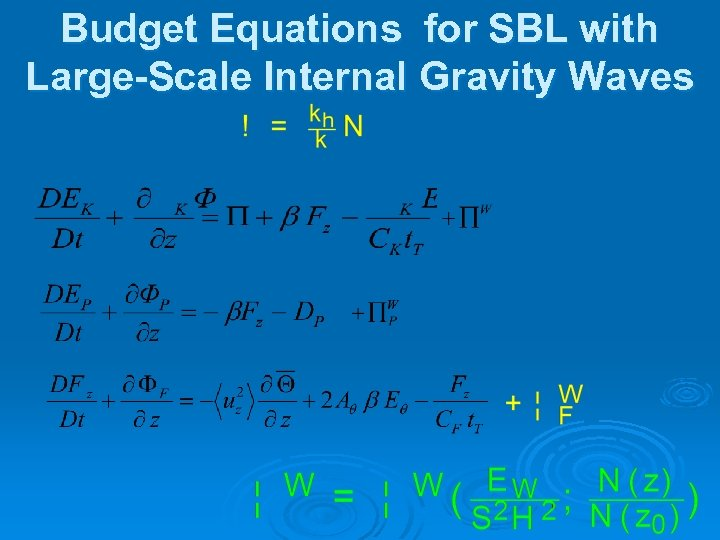Budget Equations for SBL with Large-Scale Internal Gravity Waves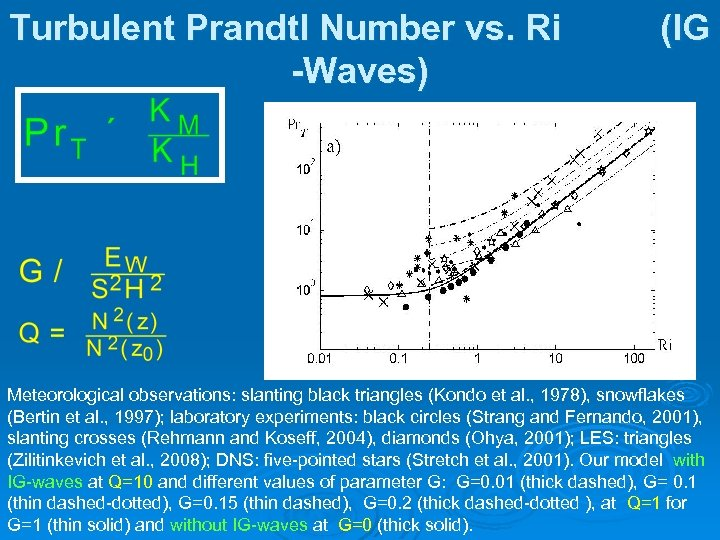Turbulent Prandtl Number vs. Ri -Waves) (IG Meteorological observations: slanting black triangles (Kondo et al. , 1978), snowflakes (Bertin et al. , 1997); laboratory experiments: black circles (Strang and Fernando, 2001), slanting crosses (Rehmann and Koseff, 2004), diamonds (Ohya, 2001); LES: triangles (Zilitinkevich et al. , 2008); DNS: five-pointed stars (Stretch et al. , 2001). Our model with IG-waves at Q=10 and different values of parameter G: G=0. 01 (thick dashed), G= 0. 1 (thin dashed-dotted), G=0. 15 (thin dashed), G=0. 2 (thick dashed-dotted ), at Q=1 for G=1 (thin solid) and without IG-waves at G=0 (thick solid).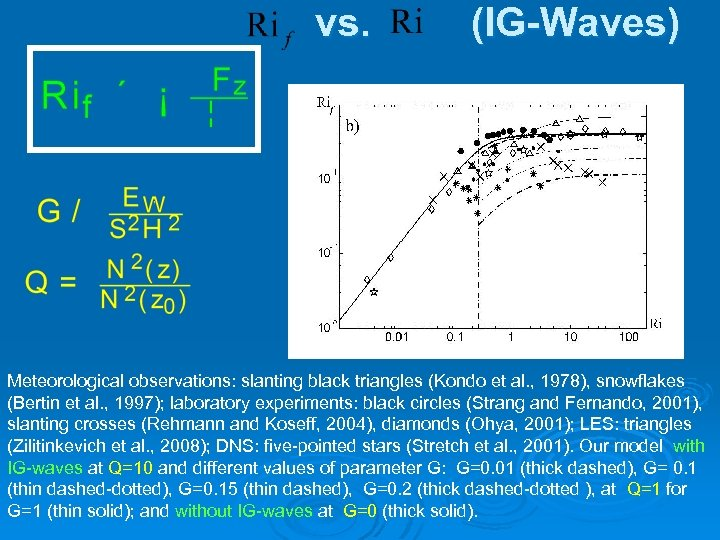vs. (IG-Waves) Meteorological observations: slanting black triangles (Kondo et al. , 1978), snowflakes (Bertin et al. , 1997); laboratory experiments: black circles (Strang and Fernando, 2001), slanting crosses (Rehmann and Koseff, 2004), diamonds (Ohya, 2001); LES: triangles (Zilitinkevich et al. , 2008); DNS: five-pointed stars (Stretch et al. , 2001). Our model with IG-waves at Q=10 and different values of parameter G: G=0. 01 (thick dashed), G= 0. 1 (thin dashed-dotted), G=0. 15 (thin dashed), G=0. 2 (thick dashed-dotted ), at Q=1 for G=1 (thin solid); and without IG-waves at G=0 (thick solid).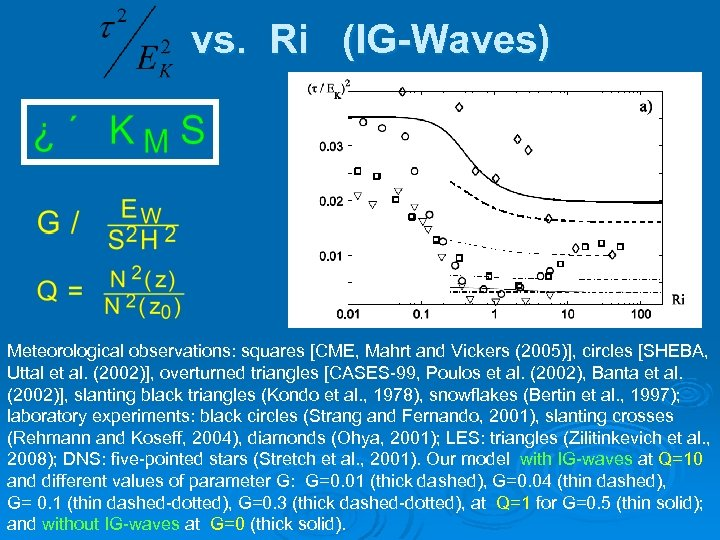vs. Ri (IG-Waves) Meteorological observations: squares [CME, Mahrt and Vickers (2005)], circles [SHEBA, Uttal et al. (2002)], overturned triangles [CASES-99, Poulos et al. (2002), Banta et al. (2002)], slanting black triangles (Kondo et al. , 1978), snowflakes (Bertin et al. , 1997); laboratory experiments: black circles (Strang and Fernando, 2001), slanting crosses (Rehmann and Koseff, 2004), diamonds (Ohya, 2001); LES: triangles (Zilitinkevich et al. , 2008); DNS: five-pointed stars (Stretch et al. , 2001). Our model with IG-waves at Q=10 and different values of parameter G: G=0. 01 (thick dashed), G=0. 04 (thin dashed), G= 0. 1 (thin dashed-dotted), G=0. 3 (thick dashed-dotted), at Q=1 for G=0. 5 (thin solid); and without IG-waves at G=0 (thick solid).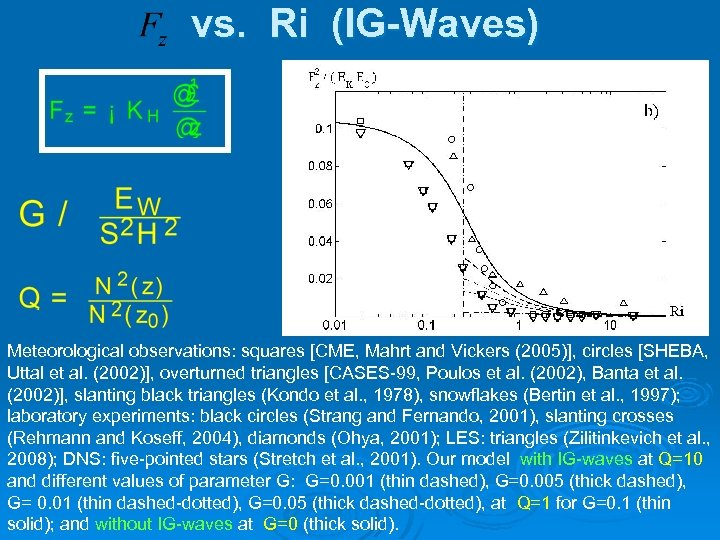vs. Ri (IG-Waves) Meteorological observations: squares [CME, Mahrt and Vickers (2005)], circles [SHEBA, Uttal et al. (2002)], overturned triangles [CASES-99, Poulos et al. (2002), Banta et al. (2002)], slanting black triangles (Kondo et al. , 1978), snowflakes (Bertin et al. , 1997); laboratory experiments: black circles (Strang and Fernando, 2001), slanting crosses (Rehmann and Koseff, 2004), diamonds (Ohya, 2001); LES: triangles (Zilitinkevich et al. , 2008); DNS: five-pointed stars (Stretch et al. , 2001). Our model with IG-waves at Q=10 and different values of parameter G: G=0. 001 (thin dashed), G=0. 005 (thick dashed), G= 0. 01 (thin dashed-dotted), G=0. 05 (thick dashed-dotted), at Q=1 for G=0. 1 (thin solid); and without IG-waves at G=0 (thick solid).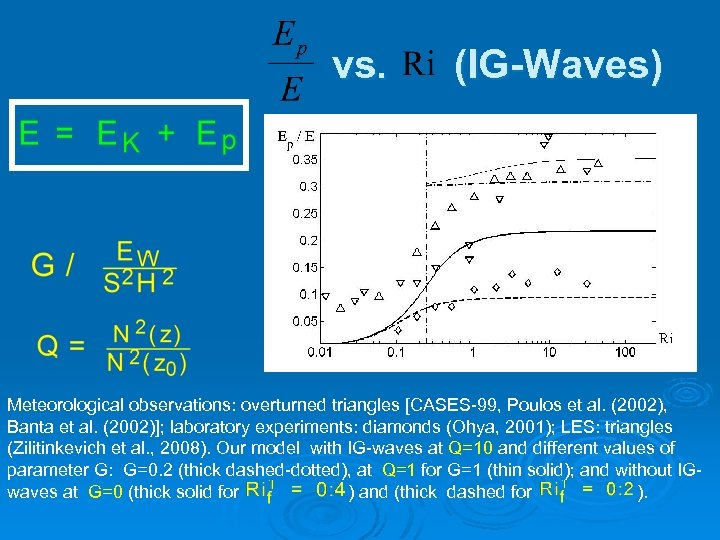vs. (IG-Waves) Meteorological observations: overturned triangles [CASES-99, Poulos et al. (2002), Banta et al. (2002)]; laboratory experiments: diamonds (Ohya, 2001); LES: triangles (Zilitinkevich et al. , 2008). Our model with IG-waves at Q=10 and different values of parameter G: G=0. 2 (thick dashed-dotted), at Q=1 for G=1 (thin solid); and without IGwaves at G=0 (thick solid for ) and (thick dashed for ).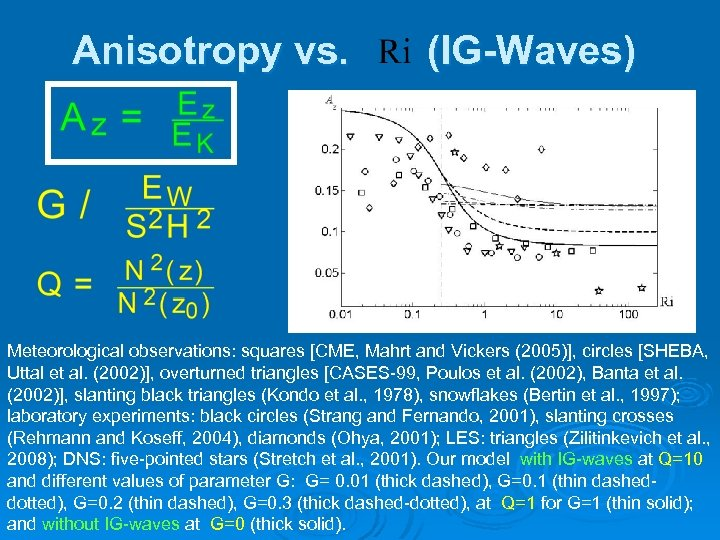Anisotropy vs. (IG-Waves) Meteorological observations: squares [CME, Mahrt and Vickers (2005)], circles [SHEBA, Uttal et al. (2002)], overturned triangles [CASES-99, Poulos et al. (2002), Banta et al. (2002)], slanting black triangles (Kondo et al. , 1978), snowflakes (Bertin et al. , 1997); laboratory experiments: black circles (Strang and Fernando, 2001), slanting crosses (Rehmann and Koseff, 2004), diamonds (Ohya, 2001); LES: triangles (Zilitinkevich et al. , 2008); DNS: five-pointed stars (Stretch et al. , 2001). Our model with IG-waves at Q=10 and different values of parameter G: G= 0. 01 (thick dashed), G=0. 1 (thin dasheddotted), G=0. 2 (thin dashed), G=0. 3 (thick dashed-dotted), at Q=1 for G=1 (thin solid); and without IG-waves at G=0 (thick solid).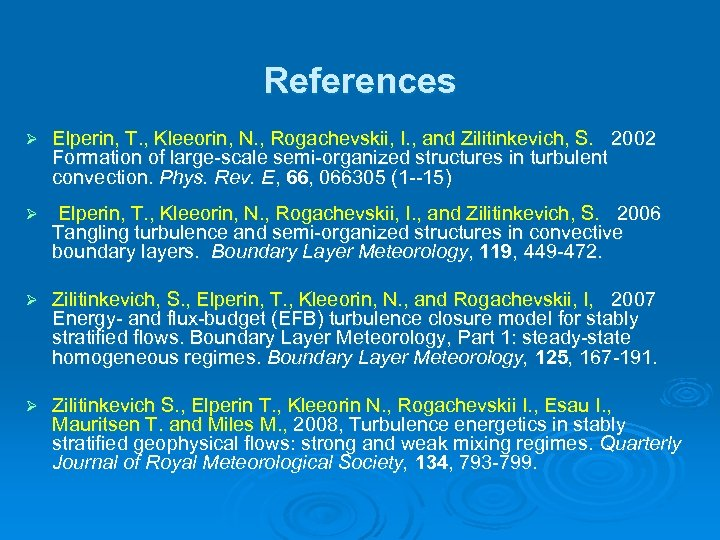References Ø Elperin, T. , Kleeorin, N. , Rogachevskii, I. , and Zilitinkevich, S. 2002 Formation of large-scale semi-organized structures in turbulent convection. Phys. Rev. E, 66, 066305 (1 --15) Ø Elperin, T. , Kleeorin, N. , Rogachevskii, I. , and Zilitinkevich, S. 2006 Tangling turbulence and semi-organized structures in convective boundary layers. Boundary Layer Meteorology, 119, 449 -472. Ø Zilitinkevich, S. , Elperin, T. , Kleeorin, N. , and Rogachevskii, I, 2007 Energy- and flux-budget (EFB) turbulence closure model for stably stratified flows. Boundary Layer Meteorology, Part 1: steady-state homogeneous regimes. Boundary Layer Meteorology, 125, 167 -191. Ø Zilitinkevich S. , Elperin T. , Kleeorin N. , Rogachevskii I. , Esau I. , Mauritsen T. and Miles M. , 2008, Turbulence energetics in stably stratified geophysical flows: strong and weak mixing regimes. Quarterly Journal of Royal Meteorological Society, 134, 793 -799.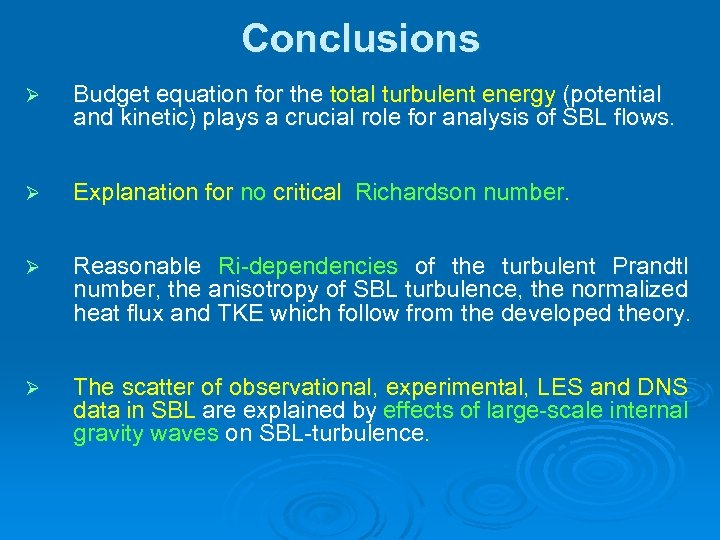Conclusions Ø Budget equation for the total turbulent energy (potential and kinetic) plays a crucial role for analysis of SBL flows. Ø Explanation for no critical Richardson number. Ø Reasonable Ri-dependencies of the turbulent Prandtl number, the anisotropy of SBL turbulence, the normalized heat flux and TKE which follow from the developed theory. Ø The scatter of observational, experimental, LES and DNS data in SBL are explained by effects of large-scale internal gravity waves on SBL-turbulence.THE END